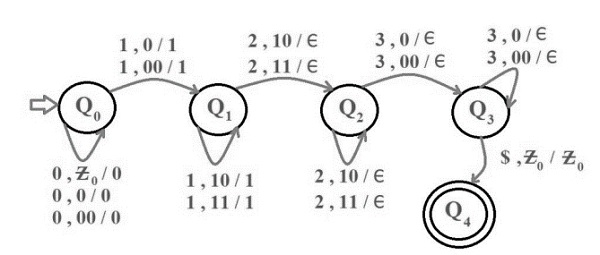# Construct a PDA for language L = {0n 1m2m3n | n>=1, m>=1}

A push down automata (PDA) can be formally described as seven tuples

(Q, Σ,S, δ,q0,I,F)

Where,

• Q is finite number of states
• Σ is input alphabet
• S is stack symbol
• Δ is the transition function: QX(Σ∪{e})XSXQ
• q0 is the initial state (q0 belongs to Q)
• I is the initial state top symbol
• F is a set of accepting states(F belongs to Q)

## Problem

Construct PDA for 0n1m2m3n where n,m≥1.

## Solution

So, the strings which are generated by the given language are −

L={0123,011223,001233….}

The number of 1’s and 3’s are same and number of 2’s and 1’s are same

Construction of PDA for given problem

The PDA is as follows −## Explanation

Step 1 − First 0’s are pushed onto the stack.

Step 2 − Next 1’s are pushed into the stack.

Step 3 − For every 2 as input a 1 is popped out of stack.

Step 4 − If some 2’s are still left and top of stack is a 0 then string is not accepted by the PDA.

Step 5 − If 2’s are finished and top of stack is a 0 then for every 3 as input equal number of 0’s are popped out of stack.

Step 6 − If the string is finished and the stack is empty then the string is accepted by the PDA. Otherwise, the string is not accepted.